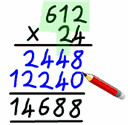# Long MultiplicationAs you can see this is an example of how to do long multiplication and i am going to walk you through it. First of all, you need to write the question down, the question that i have chosen is 612 times 24. Now that we have wrote the question down we come to the first step of long multiplication. The first step of long multiplication in my question is you do 612 times by whatever is in the ones column below 612 which is 4 and when you times all the numbers by 4 one by one you will get 2448  and you need to lay it out below the numbers you have times 4 by like it shows on the example. Secondly, you have to put a place holder zero because you are going to times 612 with whatever is on the tens column which is two but because we have done multiplying by 4 it is a twenty so we put the zero in the second column then, we need to times 612 by 2 and you should have got 12240 and it needs to be directly under the digits on top like you can see on the example question. Last but not least, you need to put a line below the second column and start doing addition (2448+12240) and the final answer is 14688 and you should really double underline your answer because that is how i have been taught in my class.